Elementary Algebra

# 4.7Graphs of Linear Inequalities

Elementary Algebra4.7 Graphs of Linear Inequalities

### Learning Objectives

By the end of this section, you will be able to:

• Verify solutions to an inequality in two variables
• Recognize the relation between the solutions of an inequality and its graph
• Graph linear inequalities

### Be Prepared 4.7

Before you get started, take this readiness quiz.

1. Solve: $4x+3>234x+3>23$.
If you missed this problem, review Example 2.73.
2. Translate from algebra to English: $x<5x<5$.
If you missed this problem, review Example 1.12.
3. Evaluate $3x−2y3x−2y$ when $x=1x=1$, $y=−2y=−2$.
If you missed this problem, review Example 1.55.

### Verify Solutions to an Inequality in Two Variables

We have learned how to solve inequalities in one variable. Now, we will look at inequalities in two variables. Inequalities in two variables have many applications. If you ran a business, for example, you would want your revenue to be greater than your costs—so that your business would make a profit.

### Linear Inequality

A linear inequality is an inequality that can be written in one of the following forms:

$Ax+By>CAx+By≥CAx+ByCAx+By≥CAx+By

where $AandBAandB$ are not both zero.

Do you remember that an inequality with one variable had many solutions? The solution to the inequality $x>3x>3$ is any number greater than 3. We showed this on the number line by shading in the number line to the right of 3, and putting an open parenthesis at 3. See Figure 4.30.

Figure 4.30

Similarly, inequalities in two variables have many solutions. Any ordered pair $(x,y)(x,y)$ that makes the inequality true when we substitute in the values is a solution of the inequality.

### Solution of a Linear Inequality

An ordered pair $(x,y)(x,y)$ is a solution of a linear inequality if the inequality is true when we substitute the values of x and y.

### Example 4.69

Determine whether each ordered pair is a solution to the inequality $y>x+4y>x+4$:

$(0,0)(0,0)$ $(1,6)(1,6)$ $(2,6)(2,6)$ $(−5,−15)(−5,−15)$ $(−8,12)(−8,12)$

### Try It 4.137

Determine whether each ordered pair is a solution to the inequality $y>x−3y>x−3$:

$(0,0)(0,0)$ $(4,9)(4,9)$ $(−2,1)(−2,1)$ $(−5,−3)(−5,−3)$ $(5,1)(5,1)$

### Try It 4.138

Determine whether each ordered pair is a solution to the inequality $y:

$(0,0)(0,0)$ $(8,6)(8,6)$ $(−2,−1)(−2,−1)$ $(3,4)(3,4)$ $(−1,−4)(−1,−4)$

### Recognize the Relation Between the Solutions of an Inequality and its Graph

Now, we will look at how the solutions of an inequality relate to its graph.

Let’s think about the number line in Figure 4.30 again. The point $x=3x=3$ separated that number line into two parts. On one side of 3 are all the numbers less than 3. On the other side of 3 all the numbers are greater than 3. See Figure 4.31.

Figure 4.31

The solution to $x>3x>3$ is the shaded part of the number line to the right of $x=3x=3$.

Similarly, the line $y=x+4y=x+4$ separates the plane into two regions. On one side of the line are points with $y. On the other side of the line are the points with $y>x+4y>x+4$. We call the line $y=x+4y=x+4$ a boundary line.

### Boundary Line

The line with equation $Ax+By=CAx+By=C$ is the boundary line that separates the region where $Ax+By>CAx+By>C$ from the region where $Ax+By.

For an inequality in one variable, the endpoint is shown with a parenthesis or a bracket depending on whether or not $aa$ is included in the solution: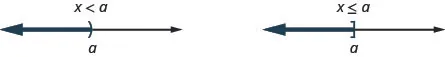Similarly, for an inequality in two variables, the boundary line is shown with a solid or dashed line to indicate whether or not it the line is included in the solution. This is summarized in Table 4.48

 $Ax+By $Ax+By≤CAx+By≤C$ $Ax+By>CAx+By>C$ $Ax+By≥CAx+By≥C$ Boundary line is not included in solution. Boundary line is included in solution. Boundary line is dashed. Boundary line is solid.
Table 4.48

Now, let’s take a look at what we found in Example 4.69. We’ll start by graphing the line $y=x+4y=x+4$, and then we’ll plot the five points we tested. See Figure 4.32.

Figure 4.32

In Example 4.69 we found that some of the points were solutions to the inequality $y>x+4y>x+4$ and some were not.

Which of the points we plotted are solutions to the inequality $y>x+4y>x+4$? The points $(1,6)(1,6)$ and $(−8,12)(−8,12)$ are solutions to the inequality $y>x+4y>x+4$. Notice that they are both on the same side of the boundary line $y=x+4y=x+4$.

The two points $(0,0)(0,0)$ and $(−5,−15)(−5,−15)$ are on the other side of the boundary line $y=x+4y=x+4$, and they are not solutions to the inequality $y>x+4y>x+4$. For those two points, $y.

What about the point $(2,6)(2,6)$? Because $6=2+46=2+4$, the point is a solution to the equation $y=x+4y=x+4$. So the point $(2,6)(2,6)$ is on the boundary line.

Let’s take another point on the left side of the boundary line and test whether or not it is a solution to the inequality $y>x+4y>x+4$. The point $(0,10)(0,10)$ clearly looks to be to the left of the boundary line, doesn’t it? Is it a solution to the inequality?

$y>x+410>?0+410>4So,(0,10)is a solution toy>x+4.y>x+410>?0+410>4So,(0,10)is a solution toy>x+4.$

Any point you choose on the left side of the boundary line is a solution to the inequality $y>x+4y>x+4$. All points on the left are solutions.

Similarly, all points on the right side of the boundary line, the side with $(0,0)(0,0)$ and $(−5,−15)(−5,−15)$, are not solutions to $y>x+4y>x+4$. See Figure 4.33.

Figure 4.33

The graph of the inequality $y>x+4y>x+4$ is shown in Figure 4.34 below. The line $y=x+4y=x+4$ divides the plane into two regions. The shaded side shows the solutions to the inequality $y>x+4y>x+4$.

The points on the boundary line, those where $y=x+4y=x+4$, are not solutions to the inequality $y>x+4y>x+4$, so the line itself is not part of the solution. We show that by making the line dashed, not solid.

Figure 4.34 The graph of the inequality $y>x+4y>x+4$.

### Example 4.70

The boundary line shown is $y=2x−1y=2x−1$. Write the inequality shown by the graph.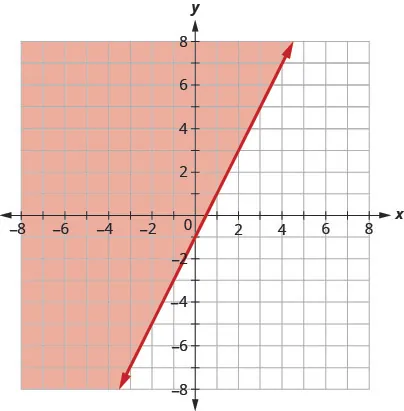### Try It 4.139

Write the inequality shown by the graph with the boundary line $y=−2x+3y=−2x+3$.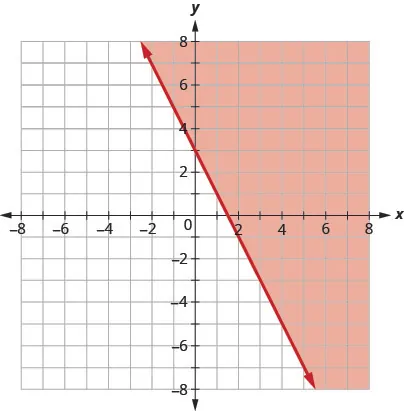### Try It 4.140

Write the inequality shown by the graph with the boundary line $y=12x−4y=12x−4$.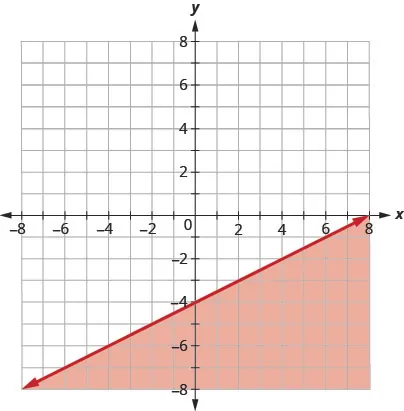### Example 4.71

The boundary line shown is $2x+3y=62x+3y=6$. Write the inequality shown by the graph.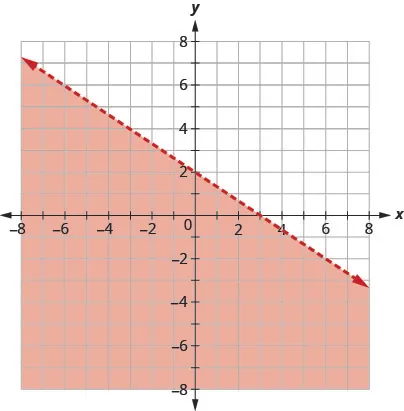### Try It 4.141

Write the inequality shown by the shaded region in the graph with the boundary line $x−4y=8x−4y=8$.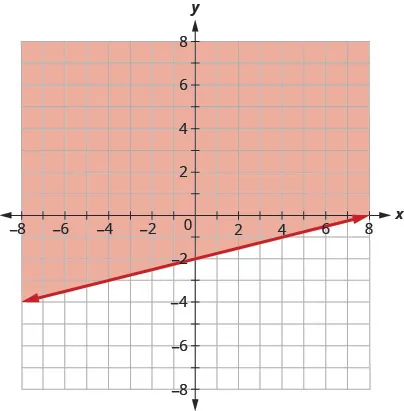### Try It 4.142

Write the inequality shown by the shaded region in the graph with the boundary line $3x−y=63x−y=6$.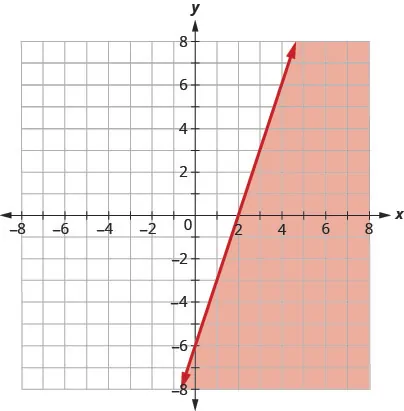### Graph Linear Inequalities

Now, we’re ready to put all this together to graph linear inequalities.

### Example 4.72

#### How to Graph Linear Inequalities

Graph the linear inequality $y≥34x−2y≥34x−2$.

### Try It 4.143

Graph the linear inequality $y≥52x−4y≥52x−4$.

### Try It 4.144

Graph the linear inequality $y<23x−5y<23x−5$.

The steps we take to graph a linear inequality are summarized here.

### How To

#### Graph a linear inequality.

1. Step 1.
Identify and graph the boundary line.
• If the inequality is $≤or≥≤or≥$, the boundary line is solid.
• If the inequality is < or >, the boundary line is dashed.
2. Step 2. Test a point that is not on the boundary line. Is it a solution of the inequality?
3. Step 3.
Shade in one side of the boundary line.
• If the test point is a solution, shade in the side that includes the point.
• If the test point is not a solution, shade in the opposite side.

### Example 4.73

Graph the linear inequality $x−2y<5x−2y<5$.

### Try It 4.145

Graph the linear inequality $2x−3y≤62x−3y≤6$.

### Try It 4.146

Graph the linear inequality $2x−y>32x−y>3$.

What if the boundary line goes through the origin? Then we won’t be able to use $(0,0)(0,0)$ as a test point. No problem—we’ll just choose some other point that is not on the boundary line.

### Example 4.74

Graph the linear inequality $y≤−4xy≤−4x$.

### Try It 4.147

Graph the linear inequality $y>−3xy>−3x$.

### Try It 4.148

Graph the linear inequality $y≥−2xy≥−2x$.

Some linear inequalities have only one variable. They may have an x but no y, or a y but no x. In these cases, the boundary line will be either a vertical or a horizontal line. Do you remember?

$x=avertical liney=bhorizontal linex=avertical liney=bhorizontal line$

### Example 4.75

Graph the linear inequality $y>3y>3$.

### Try It 4.149

Graph the linear inequality $y<5y<5$.

### Try It 4.150

Graph the linear inequality $y≤−1y≤−1$.

### Section 4.7 Exercises

#### Practice Makes Perfect

Verify Solutions to an Inequality in Two Variables

In the following exercises, determine whether each ordered pair is a solution to the given inequality.

504.

Determine whether each ordered pair is a solution to the inequality $y>x−1y>x−1$:

$(0,1)(0,1)$
$(−4,−1)(−4,−1)$
$(4,2)(4,2)$
$(3,0)(3,0)$
$(−2,−3)(−2,−3)$

505.

Determine whether each ordered pair is a solution to the inequality $y>x−3y>x−3$:

$(0,0)(0,0)$
$(2,1)(2,1)$
$(−1,−5)(−1,−5)$
$(−6,−3)(−6,−3)$
$(1,0)(1,0)$

506.

Determine whether each ordered pair is a solution to the inequality $y:

$(0,3)(0,3)$
$(−3,−2)(−3,−2)$
$(−2,0)(−2,0)$
$(0,0)(0,0)$
$(−1,4)(−1,4)$

507.

Determine whether each ordered pair is a solution to the inequality $y:

$(−3,0)(−3,0)$
$(1,6)(1,6)$
$(−6,−2)(−6,−2)$
$(0,1)(0,1)$
$(5,−4)(5,−4)$

508.

Determine whether each ordered pair is a solution to the inequality $x+y>4x+y>4$:

$(5,1)(5,1)$
$(−2,6)(−2,6)$
$(3,2)(3,2)$
$(10,−5)(10,−5)$
$(0,0)(0,0)$

509.

Determine whether each ordered pair is a solution to the inequality $x+y>2x+y>2$:

$(1,1)(1,1)$
$(4,−3)(4,−3)$
$(0,0)(0,0)$
$(−8,12)(−8,12)$
$(3,0)(3,0)$

Recognize the Relation Between the Solutions of an Inequality and its Graph

In the following exercises, write the inequality shown by the shaded region.

510.

Write the inequality shown by the graph with the boundary line $y=3x−4.y=3x−4.$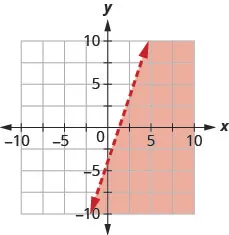511.

Write the inequality shown by the graph with the boundary line $y=2x−4.y=2x−4.$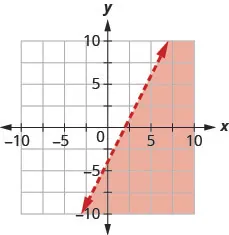512.

Write the inequality shown by the graph with the boundary line $y=−12x+1.y=−12x+1.$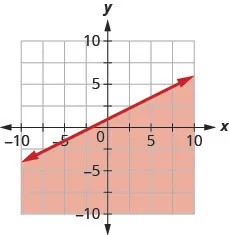513.

Write the inequality shown by the graph with the boundary line $y=−13x−2.y=−13x−2.$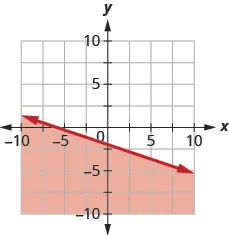514.

Write the inequality shown by the shaded region in the graph with the boundary line $x+y=5.x+y=5.$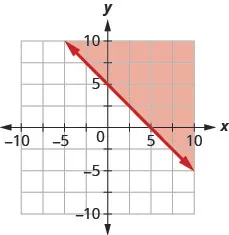515.

Write the inequality shown by the shaded region in the graph with the boundary line $x+y=3.x+y=3.$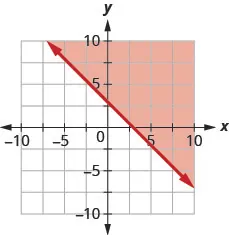516.

Write the inequality shown by the shaded region in the graph with the boundary line $2x+y=−4.2x+y=−4.$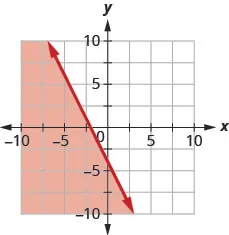517.

Write the inequality shown by the shaded region in the graph with the boundary line $x+2y=−2.x+2y=−2.$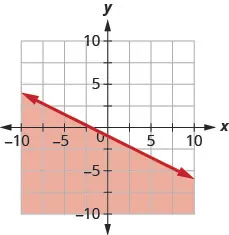518.

Write the inequality shown by the shaded region in the graph with the boundary line $3x−y=6.3x−y=6.$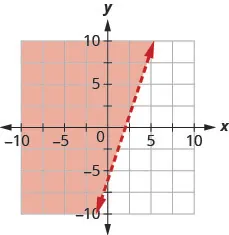519.

Write the inequality shown by the shaded region in the graph with the boundary line $2x−y=4.2x−y=4.$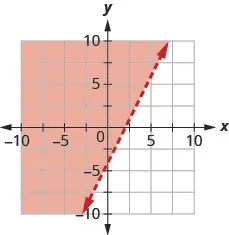520.

Write the inequality shown by the shaded region in the graph with the boundary line $2x−5y=10.2x−5y=10.$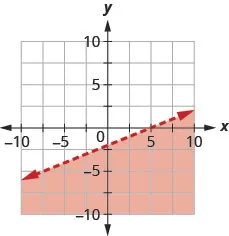521.

Write the inequality shown by the shaded region in the graph with the boundary line $4x−3y=12.4x−3y=12.$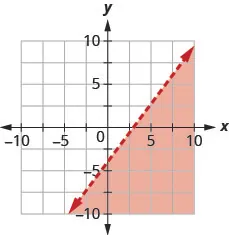Graph Linear Inequalities

In the following exercises, graph each linear inequality.

522.

Graph the linear inequality $y>23x−1y>23x−1$.

523.

Graph the linear inequality $y<35x+2y<35x+2$.

524.

Graph the linear inequality $y≤−12x+4y≤−12x+4$.

525.

Graph the linear inequality $y≥−13x−2y≥−13x−2$.

526.

Graph the linear inequality $x−y≤3x−y≤3$.

527.

Graph the linear inequality $x−y≥−2x−y≥−2$.

528.

Graph the linear inequality $4x+y>−44x+y>−4$.

529.

Graph the linear inequality $x+5y<−5x+5y<−5$.

530.

Graph the linear inequality $3x+2y≥−63x+2y≥−6$.

531.

Graph the linear inequality $4x+2y≥−84x+2y≥−8$.

532.

Graph the linear inequality $y>4xy>4x$.

533.

Graph the linear inequality $y>xy>x$.

534.

Graph the linear inequality $y≤−xy≤−x$.

535.

Graph the linear inequality $y≤−3xy≤−3x$.

536.

Graph the linear inequality $y≥−2y≥−2$.

537.

Graph the linear inequality $y<−1y<−1$.

538.

Graph the linear inequality $y<4y<4$.

539.

Graph the linear inequality $y≥2y≥2$.

540.

Graph the linear inequality $x≤5x≤5$.

541.

Graph the linear inequality $x>−2x>−2$.

542.

Graph the linear inequality $x>−3x>−3$.

543.

Graph the linear inequality $x≤4x≤4$.

544.

Graph the linear inequality $x−y<4x−y<4$.

545.

Graph the linear inequality $x−y<−3x−y<−3$.

546.

Graph the linear inequality $y≥32xy≥32x$.

547.

Graph the linear inequality $y≤54xy≤54x$.

548.

Graph the linear inequality $y>−2x+1y>−2x+1$.

549.

Graph the linear inequality $y<−3x−4y<−3x−4$.

550.

Graph the linear inequality $x≤−1x≤−1$.

551.

Graph the linear inequality $x≥0x≥0$.

#### Everyday Math

552.

Money. Gerry wants to have a maximum of $100 cash at the ticket booth when his church carnival opens. He will have$1 bills and $5 bills. If x is the number of$1 bills and y is the number of $5 bills, the inequality $x+5y≤100x+5y≤100$ models the situation. 1. Graph the inequality. 2. List three solutions to the inequality $x+5y≤100x+5y≤100$ where both x and y are integers. 553. Shopping. Tula has$20 to spend at the used book sale. Hardcover books cost $2 each and paperback books cost$0.50 each. If x is the number of hardcover books Tula can buy and y is the number of paperback books she can buy, the inequality $2x+12y≤202x+12y≤20$ models the situation.

1. Graph the inequality.
2. List three solutions to the inequality $2x+12y≤202x+12y≤20$ where both x and y are whole numbers.

#### Writing Exercises

554.

Lester thinks that the solution of any inequality with a > sign is the region above the line and the solution of any inequality with a < sign is the region below the line. Is Lester correct? Explain why or why not.

555.

Explain why in some graphs of linear inequalities the boundary line is solid but in other graphs it is dashed.

#### Self Check

After completing the exercises, use this checklist to evaluate your mastery of the objectives of this section.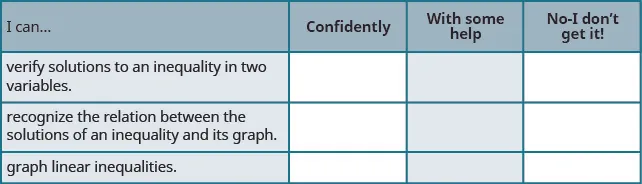What does this checklist tell you about your mastery of this section? What steps will you take to improve?

Order a print copy

As an Amazon Associate we earn from qualifying purchases.